Ten Missing number questions with solutions for ssc cgl exam

Ten Missing number questions with solutions for ssc cgl exam are discussed in this post . These questions of reasoning in latest reasoning questions with answers are very very important for upcoming competitive exams  like Bank PO , SSC CGL etc . So let us start solving and understanding these Maths logical reasoning questions with answers.

Problem # 1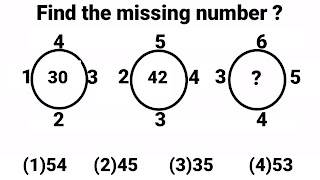Exam Cracker
There are three circles in this reasoning problem and every circle have five numbers associated to it. Out of these five numbers four are placed around the circle and fifth is in the centre of the circle. In the 3rd circle the value of question mark will be calculated using all the four associated numbers using the same formula which have been used in the 1st and 2nd circle to calculate the value of middle number.
Formula :- 3 times the sum of four numbers around the circle
3 (4 + 3 + 2  + 1 ) = 3 × 10 = 30  (  middle number in 1st figure  )
3 ( 5 + 4  + 3 + 2  ) = 3 × 14 = 42  ( middle number in 2nd figure  )
3 ( 6 + 5 + 4 + 3 ) = 3 × 18 = 54  (middle number in 3rd figure)
Option (1)54 is correct option

Problem # 2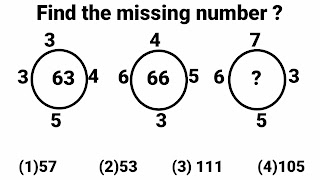Exam Cracker
There are three circles in this reasoning problem and every circle have five numbers associated to it. Out of these five numbers four are placed around the circle and fifth is in the centre of the circle. In the 3rd circle the value of question mark will be calculated using all the four associated numbers using the same formula which have been used in the 1st and 2nd circle to calculate the value of middle number.
Formula :- Left number + ( Product of remaining three numbers ) = Middle number
3 + ( 3 × 4 × 5 ) = 3 + 60 =  63 ( middle number in 1st figure  )
6 + ( 4 × 5 × 3) = 6 + 60 = 66 ( middle number in 1st figure  )
6 + ( 7 × 3 × 5 ) = 6 + 105 =  111 ( middle number in 1st figure  )
Option (1)71 is correct option

Problem # 3Exam Cracker
There are three circles in this reasoning problem and every circle have five numbers associated to it. Out of these five numbers four are placed around the circle and fifth is in the centre of the circle. In the 3rd circle the value of question mark will be calculated using all the four associated numbers using the same formula which have been used in the 1st and 2nd circle to calculate the value of middle number.

Formula :- Sum of squares of four numbers around the circle is  = Middle number

1² + 4² + 3² + 2² = 1 + 16 + 9 + 4 = 30 ( middle number in 1st figure )
2² + 5² + 4² + 3² = 4 + 25 + 16 + 9 = 54 ( middle number in 2nd figure )
3² + 6² + 5² + 4² = 9 + 36 + 25 + 16 = 86 ( middle number in 3rd figure )
Option (1)86 is correct option

Problem # 4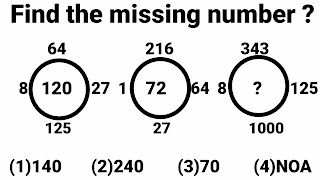Exam Cracker
There are three circles in this reasoning problem and every circle have five numbers associated to it. Out of these five numbers four are placed around the circle and fifth is in the centre of the circle. In the 3rd circle the value of question mark will be calculated using all the four associated numbers using the same formula which have been used in the 1st and 2nd circle to calculate the value of middle number.
Formula :- Product of cube root of four numbers around the circle  = Middle number
∛8 ×∛64 ×∛27 ×∛125 = 2 × 4 × 3 × 5 = 120 ( Middle number in 1st circle)
∛1 ×∛216 ×∛64 ×∛27 = 1 × 6 × 4 × 3 = 72 ( Middle number in 2nd circle)
∛8 ×∛343 ×∛125 ×∛1000 = 2 × 7 × 5 × 10 = 700 ( Middle number in 3rd circle) = The value of question mark, But 700 is not in any of three options. Therefore
Option (4)NOA is correct option

Problem # 5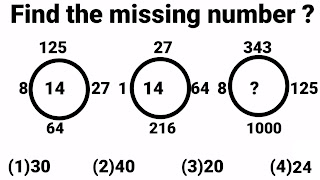Exam Cracker
There are three circles in this reasoning problem and every circle have five numbers associated to it. Out of these five numbers four are placed around the circle and fifth is in the centre of the circle. In the 3rd circle the value of question mark will be calculated using all the four associated numbers using the same formula which have been used in the 1st and 2nd circle to calculate the value of middle number.
Formula :- Sum of cube root of four numbers around the circle = Middle number
∛8 + ∛64 + ∛27 + ∛125 = 2 + 4 + 3 + 5 = 14 ( Middle number in 1st circle)
∛1 + ∛216 + ∛64 + ∛27 = 1 + 6 + 4 + 3 = 14 ( Middle number in 2nd circle)
∛8 + ∛343  + ∛125 + ∛1000 = 2 + 7 + 5 + 10 = 24 ( Middle number in 3rd circle) = The value of question mark. Therefore
Option (4)24 is correct option

Problem # 6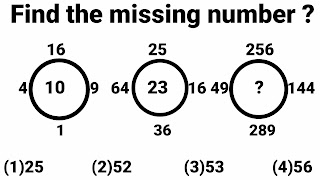Exam Cracker
There are three circles in this reasoning problem and every circle have five numbers associated to it. Out of these five numbers four are placed around the circle and fifth is in the centre of the circle. In the 3rd circle the value of question mark will be calculated using all the four associated numbers using the same formula which have been used in the 1st and 2nd circle to calculate the value of middle number.
Formula :- Sum of square root of four numbers around the circle   = Middle number
√4+ √16 + √9 + √1 = 2 + 4 + 3 + 1 = 10 ( Middle number in 1st circle)
√64+ √25 + √16 + √36 = 8 + 5 + 4 + 6 = 23 ( Middle number in 2nd circle)
√49+ √256 + √144 + √289 = 7 + 16 + 12 + 17= 52 ( Middle number in 3rd circle)
Option (2)52 is correct option

Problem # 7Exam Cracker
There are three circles in this reasoning problem and every circle have five numbers associated to it. Out of these five numbers four are placed around the circle and fifth is in the centre of the circle. In the 3rd circle the value of question mark will be calculated using all the four associated numbers using the same formula which have been used in the 1st and 2nd circle to calculate the value of middle number.
Formula :- Product of square root of four numbers around the circle  = Middle number
√4× √16 × √9 × √1 = 2 × 4 × 3 × 1= 24 ( Middle number in 1st circle)
√64+ √25 + √16 + √36 = 8 × 5 × 4 × 6= 960 ( Middle number in 2nd circle)
√9 + √100+ √16 + √121 = 3 × 10 × 4 × 11 = 1320 ( Middle number in 3rd circle)
Option (2)1320 is correct option

Problem # 8Exam Cracker
There are three circles in this reasoning problem and every circle have five numbers associated to it. Out of these five numbers four are placed around the circle and fifth is in the centre of the circle. In the 3rd circle the value of question mark will be calculated using all the four associated numbers using the same formula which have been used in the 1st and 2nd circle to calculate the value of middle number.
Formula :- Sum of square root of cube root of four numbers around the circle   = Middle number
(∛8)² + (∛125)² + (∛27)² + (∛64)² = 2² + 5² + 3² + 4² =  4 + 25 + 9 + 16 = 54 ( Middle number in 1st circle)
(∛1) ² + (∛27)² + (∛64)² + (∛216)²= 1² + 3² + 4² + 6² = 1 + 9 + 16 + 36 = 62  ( Middle number in 2nd circle)
(∛8) ² + (∛343)² + (∛125)² + (∛100)²= 2² + 7² + 5² + 10² = 4 + 49 + 25 + 100 = 178 ( Middle number in 3rd circle)  = The value of question mark. Therefore
Option (4)178 is correct option

StartExam Cracker
There are three circles in this reasoning problem and every circle have five numbers associated to it. Out of these five numbers four are placed around the circle and fifth is in the centre of the circle. In the 3rd circle the value of question mark will be calculated using all the four associated numbers using the same formula which have been used in the 1st and 2nd circle to calculate the value of middle number.
Formula :- Highest common factor of four numbers around the circle  = Middle number
HCF of  9, 12, 6 and 18 = 3
HCF of 30, 50, 60 and 20 = 10
HCF of  3, 5, 6 and 2 = 1 = The value of question mark.
Option (4)NOA is correct option

Problem # 10Exam Cracker
There are three circles in this reasoning problem and every circle have five numbers associated to it. Out of these five numbers four are placed around the circle and fifth is in the centre of the circle. In the 3rd circle the value of question mark will be calculated using all the four associated numbers using the same formula which have been used in the 1st and 2nd circle to calculate the value of middle number.
Formula :- Least common multiple of four numbers around the circle  = Middle number
LCM of  3, 2, 6 and 8 = 24
LCM of 3, 5, 6 and 10 = 30
LCM of  3, 9, 6 and 2 = 18 = The value of question mark.
Option (1)18 is correct option

Comment your valuable suggestion regarding the post "most important Reasoning questions with answers" which includes reasoning for competitive exams, circle problems, box problems, circle problems and triangles problems  for competitive exams like SSC CGL ,SSC CHSL ,CPO ,Bank exams and RRB NTPC etc which were explained in this post.
Recommended Books for you

Share:

Ten Latest Questions of missing numbers for competitive exams

Ten Latest Questions of missing numbers for competitive and Questions of missing number in reasoning for competitive exams , Bank PO, Bank clerk, SSC CGL, ssc chsl, RRB NTPC etc been discussed in post .

Ten Latest Questions of missing numbers for competitive exams

Problem # 1Exam Cracker

To find the value of question mark, 1st add both the digits of  1st number and 2nd number and then multiply the results so obtained.
Formula :- (Sum of  both digits of 1st number) × ( Sum of  both digits of 2nd numbers) .
(3 + 5 ) × (6 + 5) = 8 × 11 = 88 ( It is given)
(3 + 6 ) × (7 + 4) = 9 × 11 = 99 ( It is given)
(4 + 4 ) × (5 + 5) = 8 × 10 = 80 ( The value of question mark )
Option (4) 80 is correct option
To find the value of question mark, 1st multiply both the digits of  1st number and  add both the digits of 2nd number  and then multiply the results so obtained.
Formula :- (Product of  both digits of 1st number) × (Sum of  both digits of 2nd number) .
(2 × 7) × ( 3 + 4 ) = 14 × 7 = 98
(1 × 6 ) × ( 3 + 4 ) =  6 × 7 = 42
(2 × 8 ) × ( 3 + 3 ) = 16 × 6 = 96
Option (3)96 is correct option
In this problem all the three given numbers are nearer to the cube of any number. Hence all the given numbers can be written as the cube of some number in addition to one more mathematical operation. If we look carefully then all these given numbers can be written as the sum of a number and cube of the same number.
Formula :-  a³ + a
8³ + 8 = 512 + 8 = 520 (1st number in given problem)
9³ + 9 = 729 + 9 = 738 (2nd number in given problem)
6³ + 6 = 216 + 6 = 222 (3rd number in given problem)
7³ + 7 = 343 + 7 = 350 (4th number in given problem) and this will be the value of question mark.
Option (4)350 is correct option
In this reasoning problem 1st number (167) is associated to 43 with the help of any rule , in the same rule we have to associate 245 to a number out of four given option.
Look carefully the given numbers consists of three digits. These three digits can be utilised with the help a formula given below.
Formula :- (Left most digit) +{middle digit × right most digit}
{ 1 + ( 6 × 7) } = 1 + 42  = 43
{ 2 + ( 4 × 5) } = 2 + 20  = 22
Option (1)22 is correct option
In this reasoning problem 1st number (167) is associated to 112 with the help of some rule , With the help of same rule we have to associate 452 to a number out of four given option. Also in this problem all the  numbers consists of three digits. These three digits can be used to find the value of question mark with the help a formula given below.
Formula :- (Two Left most digits  ×  right most digit}.
In this problem both the left most digits can be taken as single unit to multiply with the 3rd number.
{ 16  × 7 } = 112
{ 45 × 2 } =  90 = ? ( The value of question mark )
Option (4)90 is correct option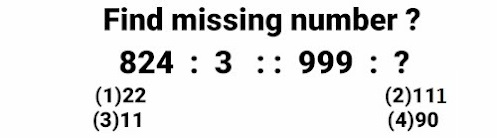Exam Cracker

In this reasoning problem 1st number (824) is associated to 3 with the help of any rule , in the same way we have to associate 999 to a number out of four given option. All the three digits of given numbers can be utilised with the help a formula given below.
Formula :- (Two Right most digits  ÷  Left most digit}
In this problem both the two right most digits can be taken as single unit to get divided with the 3rd number.
24 ÷ 8 = 3
99 ÷ 9 = 11= ? ( The value of question mark)
Option (2)11 is correct option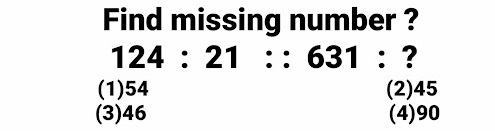Exam Cracker

This reasoning problem also consists of three digits .In this problem we have to transform 124 to 21 using a rule in the same way we have to change 631 to a number out four given options. To solve this problem means to get the 2nd number from 1st number ,take the squares of all the digits of 1st number and add all the three results so obtained.
Formula :- (1st digit)²  +  (2nd digit)² +  (3rd digit)²
1² +  2² +  4²  = 1 + 4 + 16 = 21
6² +  3² +  1² = 36 + 9 + 1 = 46 =? ( The value of question mark)
Option (3)46 is correct option
This reasoning problem also consists of three digits . In this problem we have to transform 124 to 69 using any rule in the same way we have to change 521 to a number out four given options. To solve this problem means to find the value of 2nd number in this figure , calculate  the sum of 1st digit , squares of middle digit and cube of 3rd digit .
Formula :- (1st digit)  +  (2nd digit)² + (3rd digit)³
1 +  2² +  4³  = 1 + 4 + 64 = 69
5 +   2² +  1³ = 5 + 4 + 1 = 10= ? ( The value of question mark)
Option (4)10 is correct option

Problem # 9Exam Cracker
In this reasoning problem 1st number (424) is associated to 96 with the help of any rule , in the same rule we have to associate 521 to a number out of four given option. These three digits can be utilised with the help a formula given below.
Formula :- (Two Right most digits  ×  Left most digit}
In this problem both the right most digits can be taken as single unit to multiply with the 1st number.
4 × 24 = 96
5  × 21 = 105 = ? ( The value of question mark)
Option (1)105 is correct option

Problem # 10Exam Cracker

In this reasoning problem 1st number (538) is associated to 725 with the help of any rule , in the same rule we have to associate 813 to a number out of four given option. These three digits can be utilised with the help a formula given below.

Formula :- (1st digit)  +  (2nd digit) + (3rd digit)

5  + 3 + 8 = 16
7  + 2 + 5 = 14 Sum of digits is 2 lees than above sum
8  + 1 + 3 = 12
We have to choose that option whose sum of digits must be 2 less than above sum. Therefore
7  + 1 + 2 = 10
Since all the three remaining option have total other than 10.
8 + 1 + 4 = 13
2 + 1 + 9 = 12
3 + 2 + 8 = 13 = ? ( The value of question mark)
Option (2)712 is correct option

Conclusion

Comment your valuable suggestion regarding the post most important Reasoning questions with answers which includes reasoning for competitive exams, circle problems, box problems, circle problems and triangles problems  for competitive exams like SSC CGL ,SSC CHSL ,CPO ,Bank exams and RRB NTPC etc which were explained in this post.
Recommended Books for you

Share:

Maths logical reasoning questions with Answers

Most important Reasoning questions with answers which includes circle problems, box problems, circle problems, triangles problems  for competitive exams like SSC CGL ,SSC CHSL ,CPO ,Bank exams and RRB NTPC etc have been explained in this post.

Ten Important Reasoning questions with answers for competitive exams

Problem #1

3² + 4² = 5²
➡ 9 + 16 = 25
➡ 25 = 25
L H S = R H S

6² + 8² = 10²
➡ 36 + 64 = 100
➡ 100 = 100
L H S = R H S

7² + 24² = 25²
➡ 49 + 576 = 625
➡ 625 = 625
L H S = R H S

4th Row

12² + ?² = 13²
➡ 144 + ?²  = 169
➡ ?²  = 169 -144
➡ ?²  = 25 , Taking square root on both sides
? = 5

Option (C)5 is correct option

Problem #2

1st Rectangle

{(11 + 4) × ( 9 + 5 ) } - 5  = (15 × 14) - 5 = 210 - 5 = 205

2nd Rectangle

{(21 + 1) × ( 3 + 2 ) } - 5 = (22 × 5) - 5 = 110 - 5 = 105

3rd Rectangle

{(62 + 5) × ( 1 + 1 ) } - 5  = ( 67 × 2) - 5 = 134 - 5 = 129 = ?
Option (2)129 is correct option

Problem #3

This reasoning problem consists of three triangles and every triangle have four numbers ,three around the triangle and one in the centre of the triangle.
Formula :-  Product of all the outer numbers  = Middle numbers

1st Figure

5 × 6 × 4 = 120 ⇒1 + 2 + 0 = 3 ( Middle number in 1st triangle )

2nd Figure

6 × 7 × 5 = 210  ⇒2 + 1 + 0 = 3 ( Middle number in 2nd triangle )

3rd Figure

4 × 8 × 10 = 320 ⇒3 + 2 + 0 = 5 ( Middle number in 3rd triangle )
Option (2)5 is correct option

This reasoning problem consists of three squares and every squares have four small squares , And each small square contains a number in it. If we add all the numbers in each small square then we shall have total of 35 in each small square.

1st Square

5  + 12 + 8 + 10 = 35

2nd Square

8 + 16 + 6 + 5 = 35

3rd  Square

4 + 10 + ? + 15 = 35
29 + ? = 35
? = 35 - 6
? = 6

Option (4)6 is correct option

Problem #5

HCF ( Highest common Factor ) of 4 and 8 is 4

HCF ( Highest common Factor ) of 3 and 5 is 1

HCF ( Highest common Factor ) of  6 and 12 is 6

HCF ( Highest common Factor ) of 10 and 25 is 5
Option (2)5 is correct option

Problem #6

All the numbers which are around the circle in any figure do not have any role to make the number in centre of the circle. But all the digits of the central number in 1st figure are reversed, So in same way all the digits of the central number in 2nd figure will be reversed to get the central/middle number in the 3rd figure. Hence 6543 will be the value of question mark.
Option (4)6543 is correct option

Problem #7

Sum of  all the numbers in any particular column are same for all column like

Formula :- All Numbers in any columns have equal total.

[10 + 7](1st Column) = 17 = [9 + ? + 6](2nd Column)  = [ 15 +2] = 17( 3rd Column) . Now calculating the value of ? from any two column.
→ ? = 2
Option (1)2 is correct option

Problem #8

Sum of square of three numbers in upper line is equal to the number in lower line in each figure.
Formula :-  Sum of the squares of numbers in 1st row  = Number in 2nd row

1st Figure :-

2² + 5² + 6² = 4 + 25 + 36 = 65

2nd Figure :-

6² + 8² + 9² = 36 + 64 + 81 = 81

3rd Figure :-

2² + 8² + 7² = 4 + 64 + 49 = 117
Option (2)117 is correct option

Problem #9

Sum of all the numbers in every row is 20.
2 + 3 + 7 + 8 = 20 ( Last Row )
4 + 7 + 9 = 20  ( 2nd Last Row )
11 + 9 = 20  ( 2nd Row )
Hence the value of  "?"  must be 20
Option (4)20 is correct option

Problem #10

This Reasoning question have three triangles in it, And every triangle consists of four numbers around it , The middle number in each triangle can be written using the formula given below

1st Triangle :-

7² - ( 4² + 3²) = 49 - (16 + 9 ) = 49 -25 =  24  42

2nd Triangle :-

8² - ( 6² + 3²) = 64 - (36 + 9 ) = 64 - 45 =  19  91

3rd Triangle :-

9² - ( 5² + 3²) = 81 - (25 + 9 ) = 81 - 34 =  47 74
Option (3)74 is correct option

Conclusion

Comment your valuable suggestions regarding the post most important Reasoning questions with answers which includes reasoning for competitive exams, circle problems, box problems, circle problems and triangles problems  for competitive exams like SSC CGL ,SSC CHSL ,CPO ,Bank exams and RRB NTPC etc which were explained in this post.

Share:

Most important reasoning questions for competitive exams using reasoning tricks

I have explained ten most important reasoning questions of missing number for competitive exams like ssc cgl, ssc chsl ,bank clerk and rrb ntpc . These missing number Reasoning questions have been explained in simple language and easy style.

Important reasoning questions in figure problems

Problem #1This reasoning problem consists of four figures and every figure have five numbers ,four around the figure and one in the centre of the figure. Watch carefully all the numbers which are around the central number are perfect squares. So we have to find out the square roots of these numbers and add these numbers then divide the sum with 5 to find the middle number ,

Middle number in every figure is 5th part of sum of roots of every the numbers

1st Figure

{√100 +√25 + √100 + √25 } ÷ 5 = {10 + 5 + 10 + 5 } ÷ 5  = 30 ÷ 5 = 6 ( Middle number in 1st figure )

2nd Figure

{√25 +√25 + √81 + √36 } ÷ 5 = {5 + 5 + 9 + 6 } ÷ 5 = 25 ÷ 5   = 5 ( Middle number in 2nd figure )

3rd Figure

{√25 +√25 + √25 + √25 } ÷ 5 = {5 + 5 + 5 + 5 } ÷ 5  = 20 ÷ 5 = 4 ( Middle number in 3rd figure )

4th Figure

{√36 +√49 + √9 + √16 } ÷ 5 = { 6  + 7 + 3 + 4 } ÷ 5  20 ÷ 5 = 4 ( Middle number in 4th figure ) .

Option (3)4 is correct option

Problem #2

This reasoning problem consists of three figures and every figure have five numbers ,four around the figure and one in the centre of the figure. In this reasoning problem the central/middle number is the difference of sum of the numbers on the left side and right side in each figure.

1st Figure

( 56 + 15 ) - ( 22 + 8 ) = 71 - 30 =  41 (Middle number in 1st figure)

2nd Figure

( 46 + 9 ) - ( 10 + 6 ) = 55 - 16 = 39  (Middle number in 1st figure)

3rd Figure

( 34 + 11 ) - ( 14 + 6 ) = 45 - 20 = 25  (Middle number in 3rd figure)

Option (4)25 is correct option

Problem #3

This problem figure also consists of three individual figures and every single figure have one number in the middle and six numbers in the upper and lower part of its circle . Since question mark of third figure is the the middle of circle , Hence this number in place of question mark shall be obtained from all other numbers which are in the lower and upper part of this circle.

Formula :-

All the numbers in the middle of all the figure are the 7 times of sum of all the digits written around the circle.

1st Figure

7 [0 + 6 + 4 + 5 + 3 + 1 ] = 7 × 19 =133 (Middle number in 1st circle)

2nd Figure

7 [8 + 2 + 5 + 3 + 4 + 6 ] = 7 × 28 =196 (Middle number in 2nd circle)

3rd Figure

7 [2 + 1 + 5 + 7 + 3 + 4 ] = 7 × 22 =154 (Middle number in 3rd circle)

Problem #4

This octagonal figure have eight numbers written on it. Look carefully these numbers are in increasing order starting from 3 and moving clockwise like this
3, 5 , 7 , 11 , 13 , 17, 19 , ? .

But these numbers do not form any pattern these they  neither have common difference nor have definite series pattern .
But if we take 1st number as ? ( question  mark ) and move clockwise like this
?, 3, 5 , 7 , 11 , 13 , 17, 19 .
Now we have a pattern of prime number, because 2 is 1st prime number and all other numbers are prime numbers. Therefore
2, 3, 5 , 7 , 11 , 13 , 17, 19 . are in prime number series pattern.

Option (4)2 is correct option

Problem #5

This series have seven numbers in it . And all the numbers are written in decreasing order . So we have to take the differences of two consecutive terms to proceed further.

Problem #6

This reasoning problem consists of three triangles and every triangle have four numbers associated to it. Each triangles have three numbers around it and one number is in the middle of it. So to find the middle number in each triangle multiply all the numbers around it then divide it with 10 .

1st triangle

(5 × 6 × 4 ) ÷ 10 = 120 ÷ 10 = 12  ( Middle number in 1st triangle )

2nd triangle

(6 × 7 × 5 ) ÷ 10 = 210 ÷ 10 = 21 ( Middle number in 2nd triangle )

3rd triangle

(4 × 8 × 10 ) ÷ 10 = 320 ÷ 10 = 32 ( Middle number in 3rd triangle ) .
Option (2)32 is correct option

Problem #7

This reasoning problem consists of three squares and every squares have four small squares , And each small square contains a number in it. If we add all the numbers in each small square then we shall have total of 35 in each small square.
Sum of all the numbers in each box is 35.

1st Square

3 + 17 + 4 + 11 = 35

2nd Square

2 + 16 + 7 + 10 = 35

3rd Square

6 + 13 + ? + 15 = 35
⇒34 + ? = 35
⇒ ? = 35 - 34
⇒ ? = 1
Option (4)1 is correct option
Also read these posts on Reasoning

Problem #8

( a + b )² + sq of any of two number when moving clockwise = Number in the outer part of opposite sector

( 7 + 2 )² +  7² = 81 + 49 = 130 ( Number in the outer part of opposite sector)

( 6 + 9 )² + 9² = 225 + 81 = 306 ( Number in the outer part of opposite sector)

( 3 + 4 )² + 4² = 49 + 16 = 65 ( Number in the outer part of opposite sector)

( 8 + 5 )² + 5² = 169 + 25 = 194 ( This number must be in  the outer part of opposite sector)

But  let us choose another option

( 8 + 5 )² +  8² = 169 + 64 = 233 ( Number in the outer part of opposite sector)
Hence option (4)306 is correct option

Problem  #9

This reasoning problem consists of three square and every square have five numbers ,four numbers are at the corner of each square and one in the centre of the square.
In this square every middle number is written as the difference of sum of opposite numbers.

1st Square

( 9 + 5 ) - ( 7 + 3) = 14 - 10 = 4 ( Middle number in 1st square ) .

2nd Square

( 12 + 13 ) - ( 8 + 9 ) = 25 - 17 = 8 ( Middle number in 2nd square ).

3rd Square

( 20 + 13 ) - ( 7 + 6 ) = 33 - 13 = 20 ( Middle number in 3rd square).

Option (1)20 is correct option

Problem #10

This octagonal figure have eight numbers written on it. Look carefully these numbers are not in any order
1, 2 , 4, 3 , 24 , 12 , 6, ?
These numbers are nor forming any pattern like this.
But if we consider these numbers pairwise opposite to each other like this
( 1 , 24 ), ( 2 , 12 ), ( 4 , 6 ), ( 3, ? ) and then check the multiplication/product of these paired numbers, we shall have same result every time  . Look at  here

1 × 24 = 2 × 12 = 4 × 6 = 3 × ? = 24
Hence 3 × ? = 24
? = 24 /3
? = 8

Option (4)8 is correct option.

These were the ten most important reasoning questions for competitive exams. I have tried to explain these missing number Reasoning questions in simple language and easy style. Please comment your valuable opinions regarding this post in comment box.
=============================================================